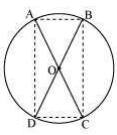Guru

# AC and BD are chords of a circle which bisect each other. Prove that (i) AC and BD are diameters; (ii) ABCD is a rectangle. Q.7

• 0

sir what is the best way for solving the question of class 9th chapter of Circles chapter of question no.1 of math of exercise 10.6 of math, what is the best way for solving the question of class 9th ncert book of question no. 7 AC and BD are chords of a circle which bisect each other. Prove that (i) AC and BD are diameters; (ii) ABCD is a rectangle

Share

1.Here chords AB and CD intersect each other at O.

Consider ΔAOB and ΔCOD,

∠AOB = ∠COD (They are vertically opposite angles)

OB = OD (Given in the question)

OA = OC (Given in the question)

So, by SAS congruency, ΔAOB ≅ ΔCOD

Also, AB = CD (By CPCT)

Similarly, ΔAOD ≅ ΔCOB

Or, AD = CB (By CPCT)

In quadrilateral ACBD, opposite sides are equal.

So, ACBD is a parallelogram.

We know that opposite angles of a parallelogram are equal.

So, ∠A = ∠C

Also, as ABCD is a cyclic quadrilateral,

∠A+∠C = 180°

⇒∠A+∠A = 180°

Or, ∠A = 90°

As ACBD is a parallelogram and one of its interior angles is 90°, so, it is a rectangle.

∠A is the angle subtended by chord BD. And as ∠A = 90°, therefore, BD should be the diameter of the circle. Similarly, AC is the diameter of the circle.

• 0